# create more fields

In order for a report to display summary figures (such as subtotals, totals, averages, and counts), you create Summary fields. A Summary field computes a statistic over all or a subset of records. In a sales report, for example, a Summary field could provide a separate total for each salesperson. (Summary fields perform calculations across a set of records, while Calculation fields perform calculations within a record.) Following are the additional fields you'll need to create for the reports in this chapter.

Field Name

Field Type

Formula or Statistic

CD_Appreciation

Calculation

CD_Current Value - CD_Amount

Stk_Dividend

Calculation

Sum (Stocks::Dividend )+Sum (Stocks::Interest)

Bond_Current Value Summary

Summary

Total of Bond_Current Value

Bond_Interest Summary

Summary

Total of Bond_Interest

Bond_Price Summary

Summary

Total of Bond_Price

CD_Amount Summary

Summary

Total of CD_Amount

CD_Appreciation Summary

Summary

Total of CD_Appreciation

CD_Current Value Summary

Summary

Total of CD_Current Value

Sav_Current Balance Summary

Summary

Total of Sav_Current Balance

Stk_Change in Value Summary

Summary

Total of Stk_Change in Value

Stk_Current Value Summary

Summary

Total of Stk_Current Value

Stk_Total Cost Summary

Summary

Total of Stk_Total Cost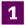If you haven't already done so, disable the Layout Preferences setting to Add newly defined fields to current layout (see page 58 for instructions).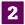Choose File > Define > Database, click the Fields tab of the Define Database dialog box, and select Main from the Table drop-down list.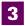Enter CD_Appreciation in the Field Name box, set Type to Calculation, and click Create. Define this formula and set the Calculation result to Number:

` CD_Current Value - CD_Amount `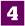Create another Calculation field named Stk_Dividend using the following formula and setting the Calculation result to Number:

` Sum (Stocks::Dividend) + Sum (Stocks::Interest) `

The fields preceded by Stocks:: must be selected from the Stocks table.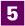To create the first Summary field, enter Bond_Current Value Summary in the Field Name box, set Type to Summary, and click Create. The Options for Summary Field dialog box appears.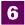Click the Total of radio button, select a field from the Available Fields list to be totaled (in this case, Bond_Current Value), and click OK.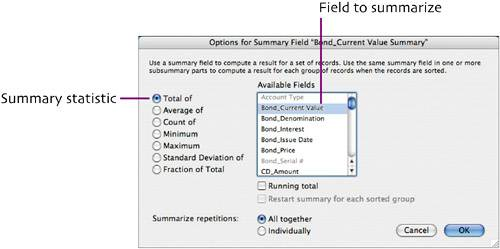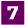Repeat Steps 5 and 6 to create the other Summary fields listed in the table on the previous page. Each Summary field uses the Total of statistic. Be sure to select the proper field to be summarized for each one.

When you're finished, click OK to close the Define Database dialog box.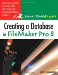Creating a Database in FileMaker Pro 8: Visual QuickProject Guide
ISBN: 0321414837
EAN: 2147483647
Year: 2005
Pages: 115

Similar book on Amazon